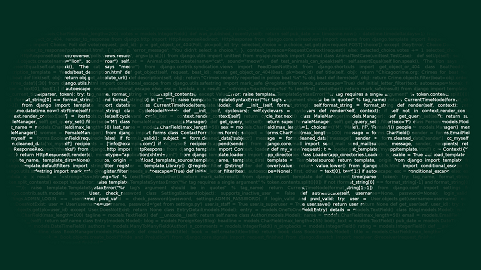# How to Combine Two Querysets in Django

Very often you may need to get results from different querysets in one place. On other words, you may want to join querysets from same or different models in Django. In this article, we will look at the different ways to combine two querysets in Django.

## How to Combine Two Querysets in Django

Here are the different ways to combine two querysets in Django. Let us say you have the following two models in Django.

```class Post(models.Model):
title = models.CharField(max_length=255)
content = models.TextField(blank=True)
published = models.DateField(blank=True)
author = models.CharField(max_length=255)

class Article(models.Model):
title = models.CharField(max_length=255)
content = models.TextField(blank=True)
published = models.DateField(blank=True)
author = models.CharField(max_length=255)```

Let us say you have the following three querysets generated from above models, that you want to combine.

```>>> q1 = Post.objects.filter(id__gte=5)
>>> q2 = Article.objects.filter(id__lte=9)
>>> q3 = Post.objects.filter(id__lte=2)```

## 1. Using Union operator

If both your querysets belong to the same model, such as q1 and q3 above, then you can use union operator | to easily combine those querysets. Here is an example to do it.

`q13 = q1 | q3`

You can use the union operator to combine two or more querysets as shown below.

`combined_result= q1 | q3 | q4 ...`

## 2. Using itertools

itertools provides chain method that allows you to easily combine two or more querysets from same or different models.

Here is an example to combine q1 and q2 querysets defined above using itertools.

````from itertools import chain `
combined`_list = list(chain(q1,q2))````

You just need to mention the querysets you want to combine in a comma-separated manner in chain function.

You can also use it to combine more than two querysets.

`combined`_list = list(chain(q1, q2, q3))``

As mentioned earlier, it is very easy to combine querysets in Django whether they belong to same or different models.